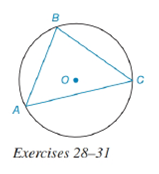Chapter 6.4, Problem 28EElementary Geometry For College St...

7th Edition
Alexander + 2 others
ISBN: 9781337614085

Solutions

Chapter
SectionElementary Geometry For College St...

7th Edition
Alexander + 2 others
ISBN: 9781337614085
Textbook Problem

Triangle A B C is inscribed in circle O ; A B = 5 , B C = 6 , and A C = 7 .a) Which is the largest minor arc of ⊙ O : A B ⌢ , B C ⌢ , or A C ⌢ ?b) Which side of the triangle is nearest point O ?To determine

(a)

To find:

The largest minor arc.

Explanation

Given:

Triangle ABC is inscribed in circle O; AB=5, BC=6, and AC=7.

The figure given below.

Theorem Used:

In a circle (or in congruent circles) containing two unequal chords, the longer chord corresponds to the greater minor arc.

Calculation:

Using the theorem,

Since the chords AB=5, BC=6, and AC=7 are unequal and AB<BC<AC.

To determine

(b)

To find:

The side of the triangle that is nearest point O.

Still sussing out bartleby?

Check out a sample textbook solution.

See a sample solution

The Solution to Your Study Problems

Bartleby provides explanations to thousands of textbook problems written by our experts, many with advanced degrees!

Get Started

In Exercises 107-120, factor each expression completely. 109. 4a2 b2

Applied Calculus for the Managerial, Life, and Social Sciences: A Brief Approach

Can the vectors in Problems 1-4 be probability vectors? If not, why? 4.

Mathematical Applications for the Management, Life, and Social Sciences

Sketch the graphs of the equations in Exercises 512. x+y=1

Finite Mathematics and Applied Calculus (MindTap Course List)

Evaluate the integral, if it exists. sintcostdt

Single Variable Calculus: Early Transcendentals